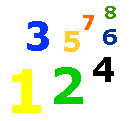# Rounding Numbers

Rounding Numbers Worksheet - Math Lessons & Tests - My Schoolhouse - Online Learning

Rounding numbers is a quick way to get an estimate of the number of things you might need or have available.

Let's say you have 97 stickers and 275 marbles.

You might say you have about 100 stickers and about 300 marbles.  This is called rounding numbers.

To round a number, you look to the first digit to the right of the digit you want to round.  If the digit is five or more, you round up.  If the digit is less than five, you round down.  In the number 27, if you want to round to the nearest ten, you look at the 7.  The 7 is 5 or greater, so you round up.

27 rounded to the nearest ten is 30.

In the number 239, if you want to round to the nearest hundred, you look at the 3.

239 rounded to the nearest hundred is 200 because 3 is less than 5.

REMEMBER: IF THE DIGIT IS 5 OR MORE, ALWAYS ROUND UP.  IF THE DIGIT IS 4 OR LESS, ALWAYS ROUND DOWN.

You can round numbers to the nearest ten.  Is the digit to the right of the tens place value 5 or greater or less than 5?

Let's take the number 23.  Is it closer to 20 or 30?

23 is rounded to 20 because the 3 is less than five.

You can round numbers to the nearest hundred.  Is the digit to the right of the hundreds place value 5 or greater or less than 5?

Let's take the number 262.  Is it closer to 200 or 300?

262 is rounded to 300.

You can round numbers to the nearest thousand.  Is the digit to the right of the thousands place value 5 or greater or less than 5?

Let's take the number 7,257.  Is it closer to 7,000 or 8,000?

7,257 is rounded to 7,000.

Practice rounding numbers.  Fill in the boxes.

Round to the tens place value.

 Number Rounded Number 27 36 12 82 47 59 71 68

Round to the hundreds place value.

 Number Rounded Number 234 689 557 131 492 325 777

Round to the thousands place value.

 Number Rounded Number 3,277 1,929 5,102 2,289 7,711 6,378 4,412

 Thank You for using My Schoolhouse